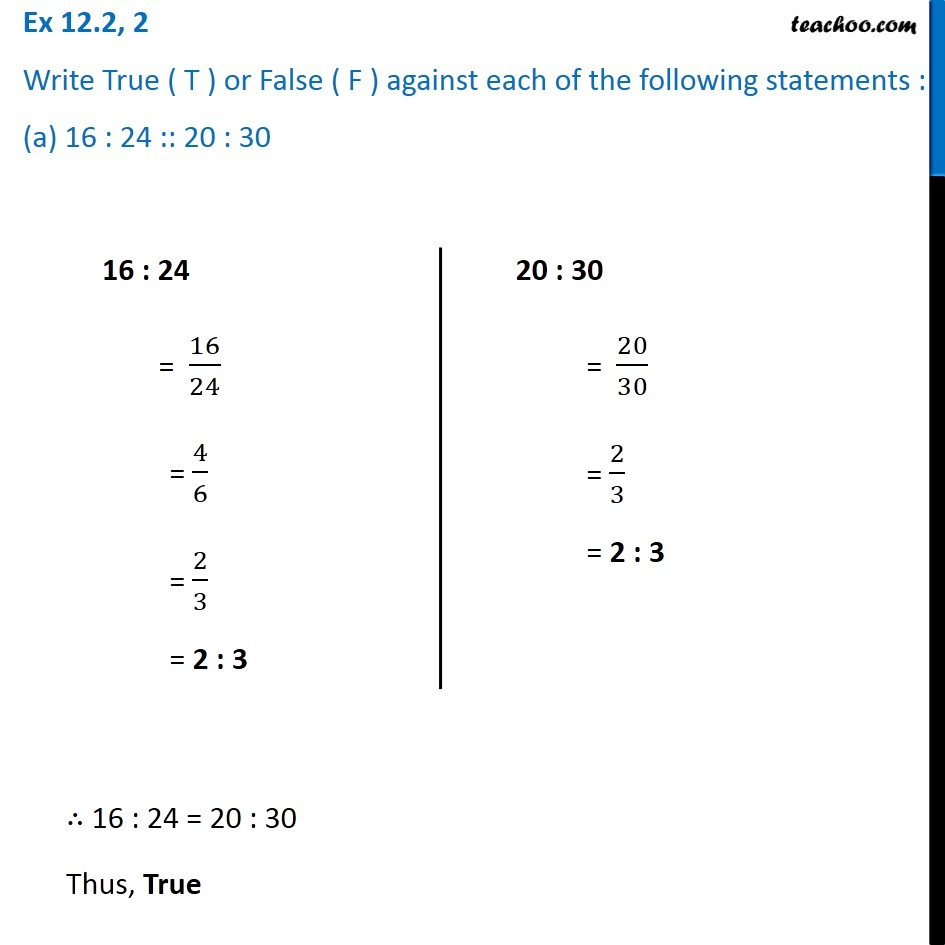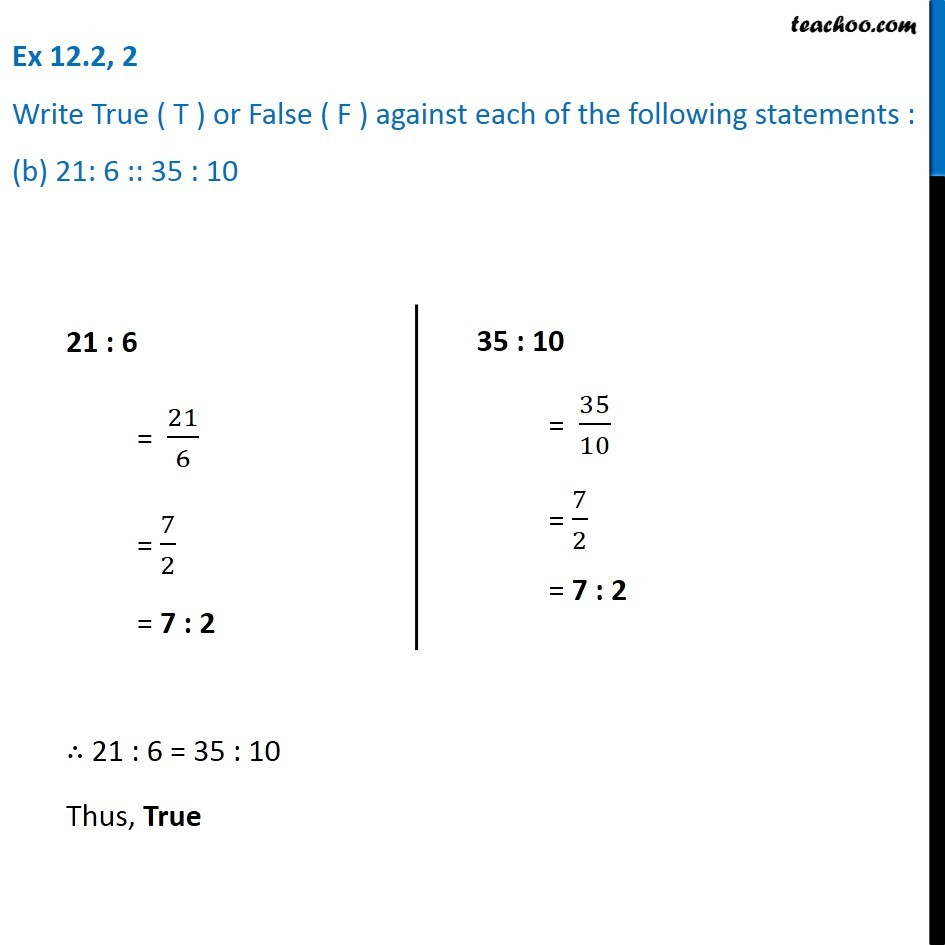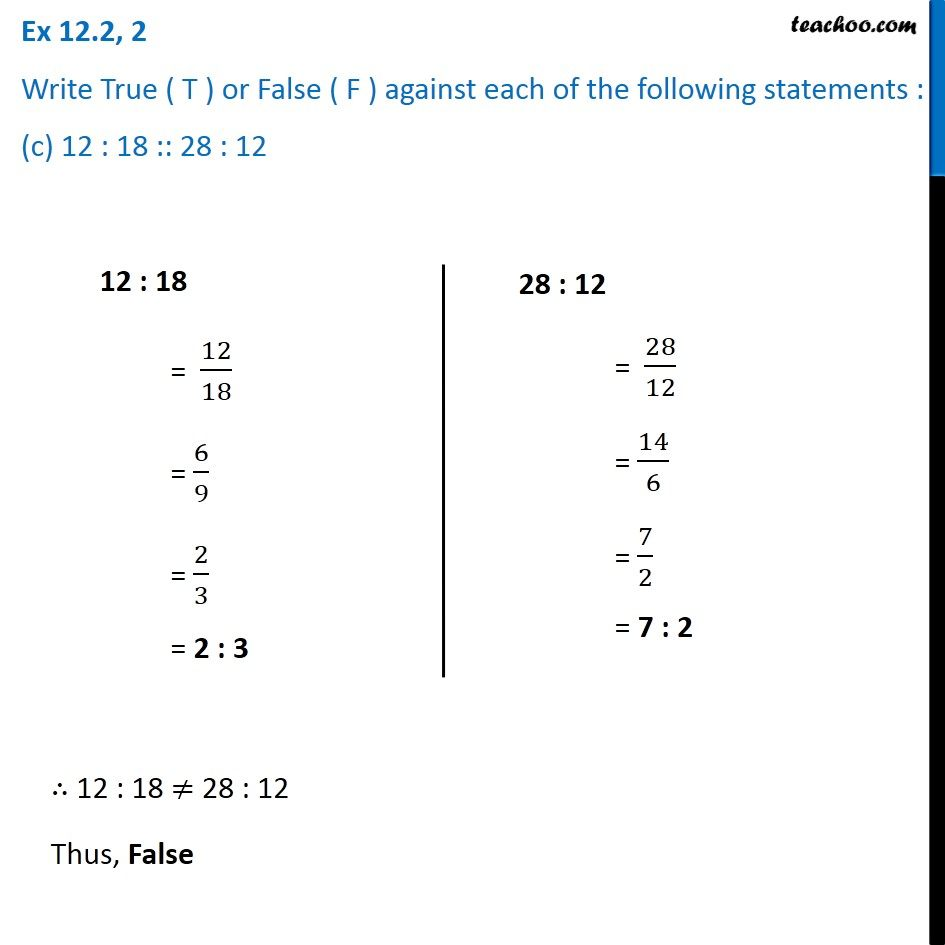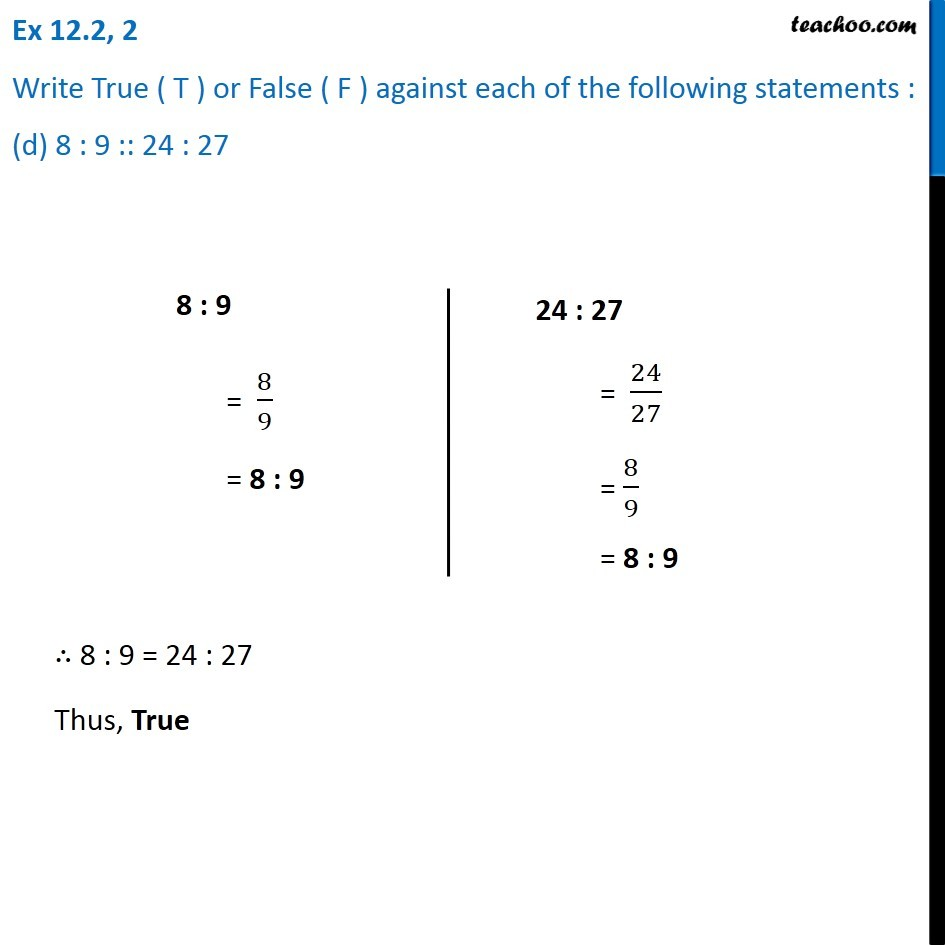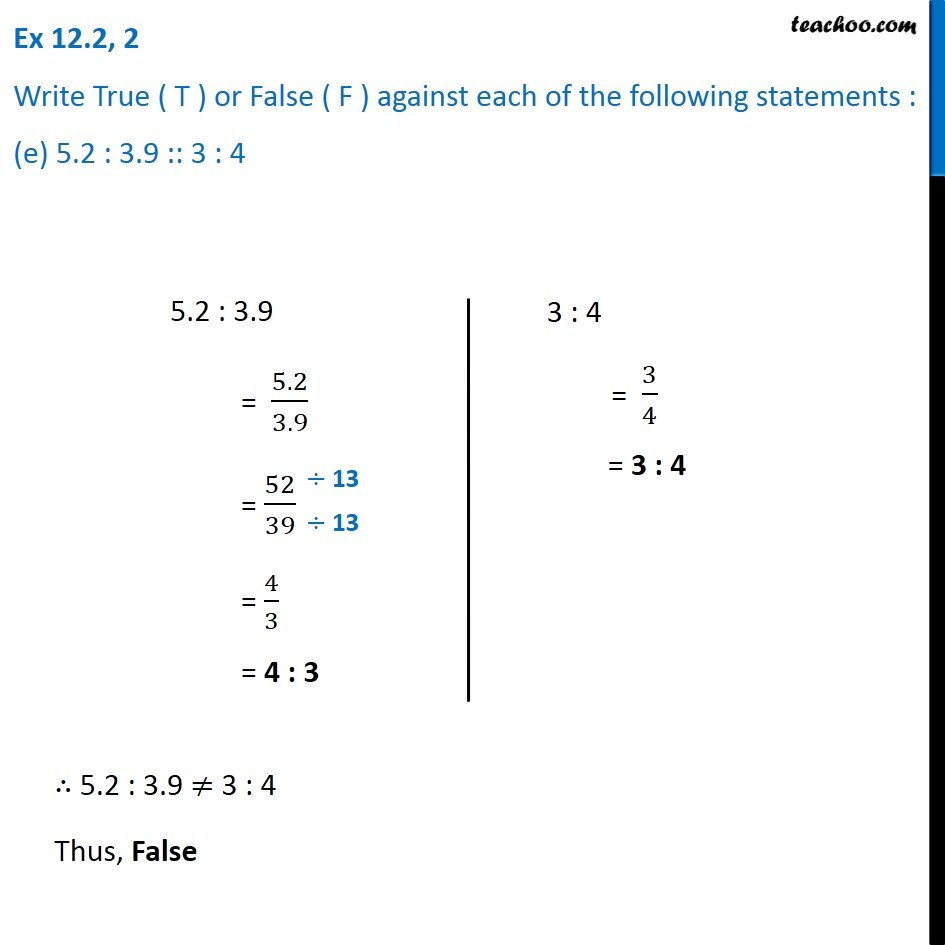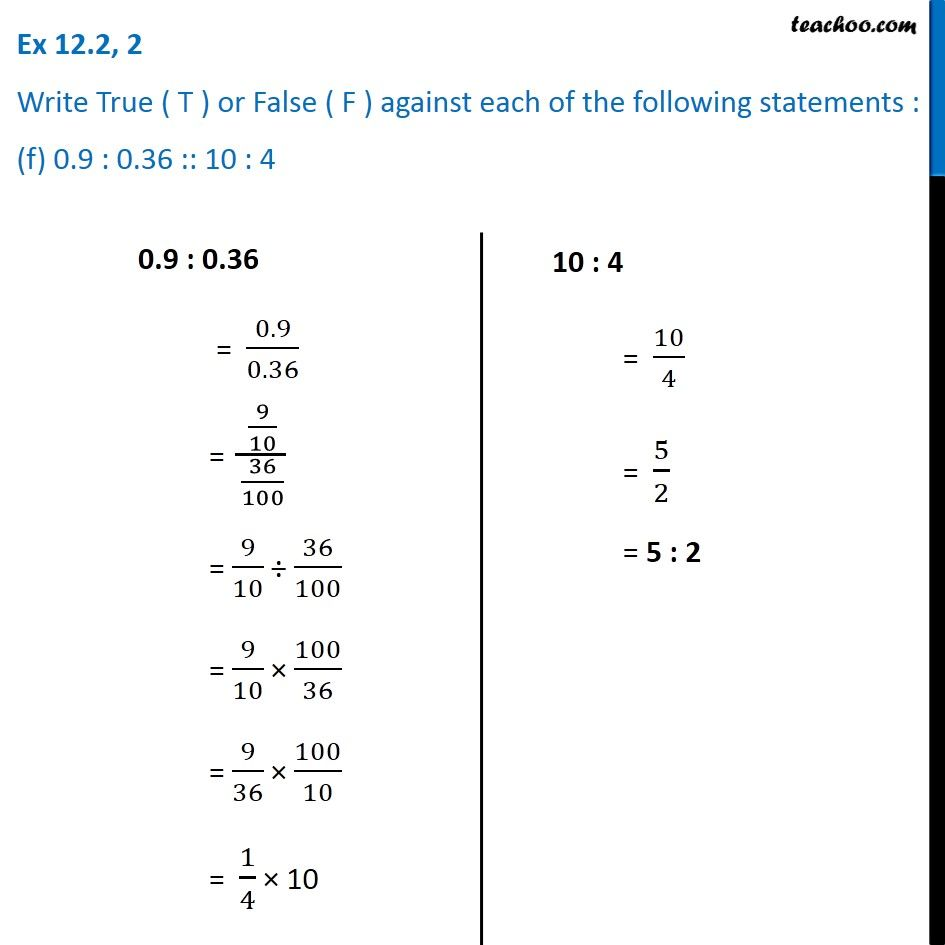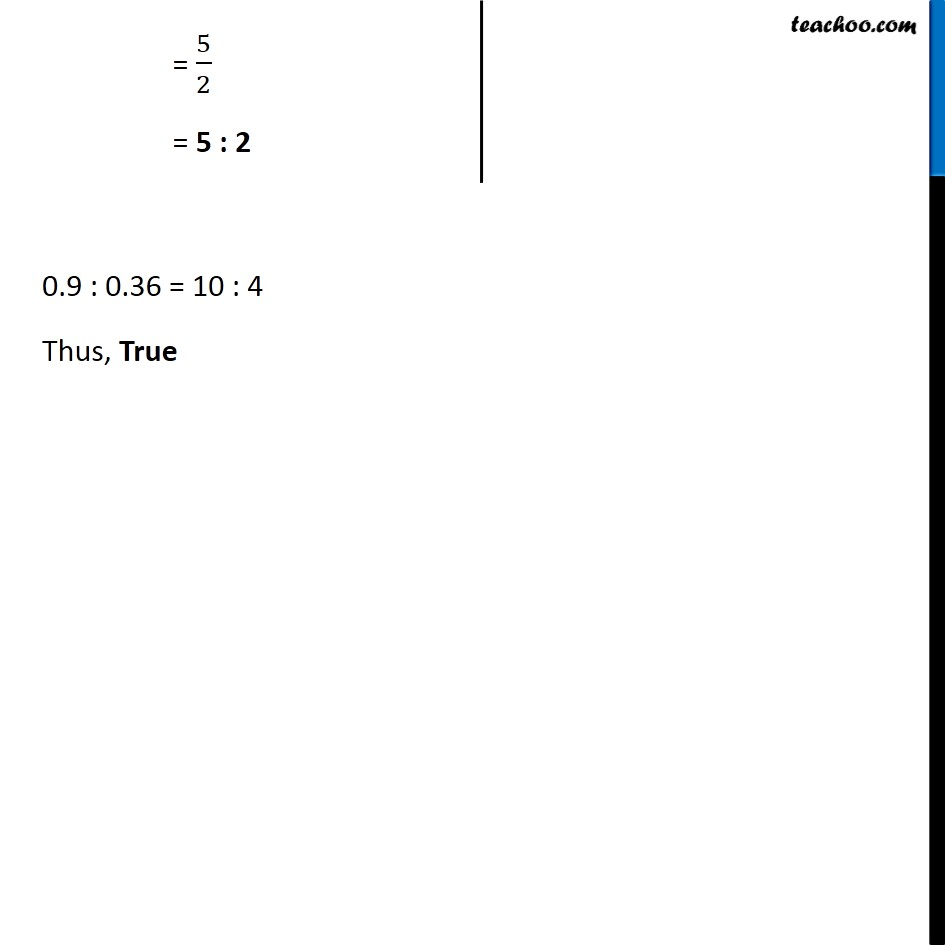Subscribe to our Youtube Channel - https://you.tube/teachoo

1. Chapter 12 Class 6 Ratio And Proportion
2. Serial order wise
3. Ex 12.2

Transcript

Ex 12.2, 2 Write True ( T ) or False ( F ) against each of the following statements : (a) 16 : 24 :: 20 : 30 16 : 24 = 16/24 = 4/6 = 2/3 = 2 : 3 20 : 30 = 20/30 = 2/3 = 2 : 3 ∴ 16 : 24 = 20 : 3021 : 6 = 21/6 = 7/2 = 7 : 2 Thus, True Ex 12.2, 2 Write True ( T ) or False ( F ) against each of the following statements : (b) 21: 6 :: 35 : 1021 : 6 = 21/6 = 7/2 = 7 : 2 35 : 10 = 35/10 = 7/2 = 7 : 2∴ 21 : 6 = 35 : 10 Thus, True Ex 12.2, 2 Write True ( T ) or False ( F ) against each of the following statements : (c) 12 : 18 :: 28 : 1212 : 18 = 12/18 = 6/9 = 2/3 = 2 : 3 28 : 12 = 28/12 = 14/6 = 7/2 = 7 : 2 ∴ 12 : 18 ≠ 28 : 12 Thus, False Ex 12.2, 2 Write True ( T ) or False ( F ) against each of the following statements : (d) 8 : 9 :: 24 : 278 : 9 = 8/9 = 8 : 9 24 : 27 = 24/27 = 8/9 = 8 : 9 ∴ 8 : 9 = 24 : 27 Thus, True Ex 12.2, 2 Write True ( T ) or False ( F ) against each of the following statements : (e) 5.2 : 3.9 :: 3 : 45.2 : 3.9 = 5.2/3.9 = 52/39 = 4/3 = 4 : 3 3 : 4 = 3/4 = 3 : 4 ∴ 5.2 : 3.9 ≠ 3 : 4 Thus, False Ex 12.2, 2 Write True ( T ) or False ( F ) against each of the following statements : (f) 0.9 : 0.36 :: 10 : 40.9 : 0.36 = 0.9/0.36 = (9/10)/(36/100) = 9/10 ÷ 36/100 = 9/10 × 100/36 = 9/36 × 100/10 = 1/4 × 10 10 : 4 = 10/4 = 5/2 = 5 : 2 = 5/2 = 5 : 2 0.9 : 0.36 = 10 : 4 Thus, True

Ex 12.2